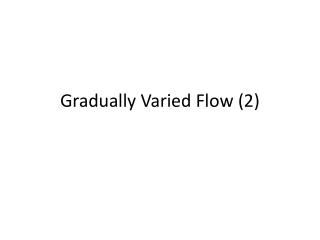DownloadDownload PresentationTélécharger la présentation- - - - - - - - - - - - - - - - - - - - - - - - - - - E N D - - - - - - - - - - - - - - - - - - - - - - - - - - -
Presentation Transcript

2. Equations • Bottom slope • Mild if (subcritical); • Steep if (supercritical); and • Critical if • Mild and steep slopes (3 Zones); the rest slopes have only two regions • The upper line is NDL if the channel bottom slope is mild, and the upper line is CDL if the bottom slope is steep.

3. Remarks • For a water surface profile in a channel with mild slope then we infer that • The flow depth, , in the three zones is classified as follows: • Zone 1: ; • Zone 2: ; and • Zone 3:

4. Profiles • (M1 Profile): since it is mild slope then the upper line is NDL. Since in Zone 1, . since • This means that y increases with distance x

5. Profiles • (M2 Profile): in this case since . because • This means, decreases as increases

6. Profiles • (M3 Profile): in this case since . because • This means, increases as increases

7. Real-life cases of water surface profiles (Choudhry, 2008)

8. Example (1) (Choudhry, 2008)

9. Example (2): Consider two different cases of gate opening (Choudhry, 2008)